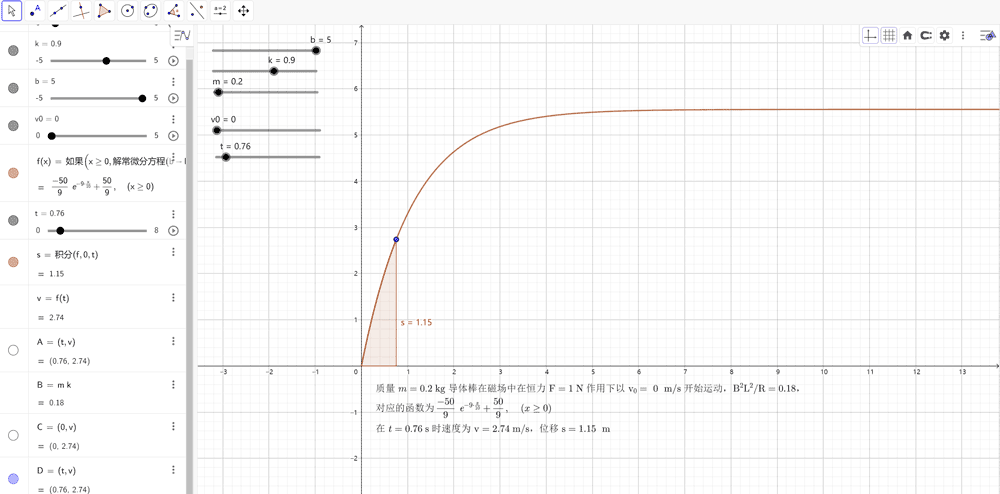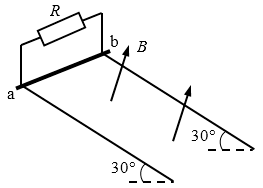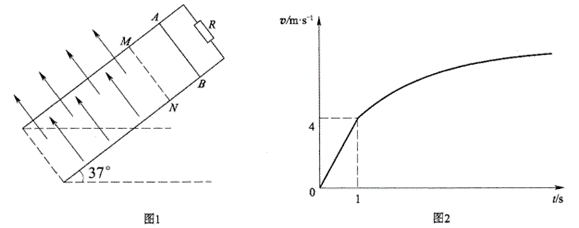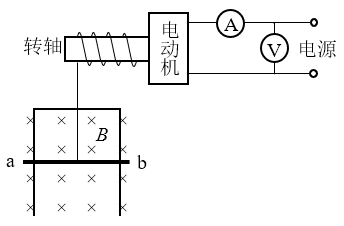# 使用 Geogebra 解常微分方程

## 1、电磁感应中恒力作用下物体做切割磁感线运动的分析

### 例 1电阻可忽略的光滑平行金属导轨长 s = 1.15 m，两导轨间距 L = 0.75 m，导轨倾角为 30°，导轨上端 ab 接一阻值 R = 1.5 Ω 的电阻，磁感应强度 B = 0.8 T 的匀强磁场垂直轨道平面向上。阻值 r = 0.5 Ω，质量 m = 0.2 kg 的金属棒与轨道垂直且接触良好，从轨道上端 ab 处由静止开始下滑至底端，在此过程中金属棒产生的焦耳热 Qr = 0.1 J。（取 g = 10m/s2）求：

（1）金属棒在此过程中克服安培力的功 W

（2）金属棒下滑速度 v = 2 m/s 时的加速度 a

（3）为求金属棒下滑的最大速度 vm，有同学解答如下：由动能定理 WW = $$\frac{1}{2}$$mvm2，……。由此所得结果是否正确？若正确，说明理由并完成本小题；若不正确，给出正确的解答。

【参考答案】（1）W = 0.4 J              （2）a = 3.2 m/s2           （3）vm =  2.74 m/s

【分析】

W = Q = QR + Qr = 0.4 J

mgssin30° − Q = $$\frac{1}{2}$$mvm2

vm = $$\sqrt {2gs\sin 30^\circ - \frac{{2Q}}{m}}$$ = $$\sqrt {2 \times 10 \times 1.15 \times 0.5 - \frac{{2 \times 0.4}}{{0.2}}}$$ m/s = 2.74 m/s

【微积分解法】

mgsinθ − $$\frac{{{B^2}{L^2}v}}{{R + r}}$$ = ma

a = 5 − 0.9v

$\frac{{\frac{{{\rm{d}}s}}{{{\rm{d}}t}}}}{{\frac{{{\rm{d}}v}}{{{\rm{d}}t}}}} = \frac{{{\rm{d}}s}}{{{\rm{d}}v}} = \frac{v}{a} = \frac{v}{{5 - 0.9v}}$

ds = $$\frac{v}{{5 - 0.9v}}$$ dv，初始条件为 s|v = 0 = 0

s = $$\frac{1}{0.81}$$（− 0.9v − 5ln（− 0.9v + 5）+ 5ln5）

s = 1.15 m 代入以上函数可解得：v = 2.74 m/s。

$$\frac{{{\rm{d}}v}}{{{\rm{d}}t}}$$ = a = 5 − 0.9vv|t = 0 = 0

t = $$\frac{10}{9}$$ [ln5 − ln(−0.9v + 5)]

v ＝ $$\frac{50}{9}$$（1 − e−0.9t

v = 2.74 m/s 代入以上函数，可求得 t = 0.75515 s。

$$\frac{{{\rm{d}}s}}{{{\rm{d}}t}}$$ = v = $$\frac{50}{9}$$（1 − e−0.9t），s|t = 0 = 0

s = $$\frac{{450t + 500{e^{ - 0.9t}} - 500}}{{81}}$$

s = 1.15 m 代入以上函数，可求得 t = 0.75484 s。数据是自洽的

## 2、使用 Geogebra 解常微分方程

a = 5 − 0.9v

$$\frac{{{\rm{d}}v}}{{{\rm{d}}t}}$$ = a = 5 − 0.9vv|t = 0 = 0

5 − 0.9v 为一线性函数，令 k = 0.9，b = 5，v0 = 0，这样就可以在 Geogebra 中动态设置这些变量，解决其他类似的问题。关键步骤如下：

1、设置对应 kbv0 变量的滑动条，设置表示时间 t 的滑动条；

2、输入 “f(x) = 解常微分方程(b – ky,(0,v0))”，这样就能绘制出 vt 图像，并显示求解结果 “f(x) ＝− $$\frac{50}{9}$$ e−0.9t + $$\frac{50}{9}$$”，这个结果与前面解析求解的结果是相同的。

3、输入 “s = 积分(f,0,t)”，这样就可以通过 vt 图像面积求出对应位移。## 3、使用 Geogebra 快速验证相关问题

### 例 2光滑平行金属导轨长 L = 2 m，二导轨间距 d = 0.5 m，轨道平面与水平面的夹角为 θ = 30°，导轨上端接一阻值为 R = 0.5 Ω 的电阻，其余电阻不计，轨道所在空间有垂直轨道平面的匀强磁场，磁感应强度 B = 1 T。有一不计电阻的金属棒 ab 的质量 m = 0.5 kg，放在导轨最上端，如图所示。当 ab 棒从最上端由静止开始自由下滑，到达底端脱离轨道时，电阻 R 上产生的热量为 Q = 1 J，求：

（1）当棒的速度为 v = 2 m/s 时，它的加速度是多少？

（2）棒下滑的最大速度是多少？

（3）棒下滑过程中通过电阻 R 的最大电流是多少？

【参考解答】（1）a = 3 m/s2        （2）vm = 4 m/s      （3）Im = 4 A

【验证】

### 例 3如图甲所示，MN、PQ 为间距 L = 0.5 m 足够长的平行导轨，NQ⊥MN，导轨的电阻均不计。导轨平面与水平面间的夹角 θ = 37°，NQ 间连接有一个 R = 4 Ω 的电阻。有一匀强磁场垂直于导轨平面且方向向上，磁感应强度为 B0 = 1 T。将一根质量为 m = 0.05 kg 的金属棒 ab 紧靠 NQ 放置在导轨上，且与导轨接触良好。现由静止释放金属棒，当金属棒滑行至 cd 处时达到稳定速度，已知在此过程中通过金属棒截面的电量 q = 0.2 C，且金属棒的加速度 a 与速度 v 的关系如图乙所示，设金属棒沿导轨向下运动过程中始终与 NQ 平行。（取 g = 10 m/s2，sin37° = 0.6，cos37° = 0.8）。求：

（1）金属棒与导轨间的动摩擦因数 μ

（2）cd 离 NQ 的距离 s

（3）金属棒滑行至 cd 处的过程中，电阻 R 上产生的热量。

【参考解答】（1）μ =  0.5  （2）s = 2 m  （3）QR =  0.08 J

【验证】

a = 2 – v

s =− v − 2ln（− v + 2）+ 2ln2

v = 2 m/s 代入上式，出现 ln0 无解！那是因为根据对应的速度时间函数 v = −2et + 2（过程略），理论上需要经过无穷大的时间才能达到稳定速度，对应的位移也是无穷大。

### 例 4（1）求金属棒与导轨间的动摩擦因数；

（2）求金属棒在磁场中能够达到的最大速率；

（3）已知金属棒从进入磁场到速度达到 5 m/s 时通过电阻的电荷量为 1.3 C，求此过程中电阻产生的焦耳热。

【参考解答】（1）0.25         （2）8 m/s              （3）2.95 J

【验证】

va 的关系式：a = 4 – 0.5v

## 4、电磁感应中恒功率作用下物体做切割磁感线运动的分析

### 例 5如图所示，电动机通过其转轴上的绝缘细绳竖直向上牵引一根原来静止的长为 L = 1 m、质量 m = 0.1 kg导体棒 ab，导体棒紧贴在竖直放置、电阻不计的金属框架上，导体棒的电阻 R = 1 Ω，磁感强度 B = 1 T 的匀强磁场方向垂直于导体框架所在平面，当导体棒在电动机牵引下上升 h = 3.8 m 时，获得稳定速度，此过程导体棒产生热量 Q = 2 J。电动机工作时，输出功率 P = 6 W 恒定，不计一切摩擦，求：

（1）导体棒所达到的稳定速度是多少？

（2）导体棒从静止到达稳定速度的时间是多少？

【验证】

【微积分求解】

$\frac{{{\rm{d}}v}}{{\frac{{60}}{v} - 10v - 10}} = {\rm{d}}t$

$- \frac{v}{{10{v^2} + 10v - 60}}{\rm{d}}v = {\rm{d}}t$

$\int {\frac{x}{{a{x^2} + bx + c}}dx} = \frac{1}{{2a}}\ln \left| {a{x^2} + bx + c} \right| - \frac{b}{{2a}}\int {\frac{dx}{{a{x^2} + bx + c}}}$

$\int {\frac{{dx}}{{a{x^2} + bx + c}}} = \left\{ {\begin{array}{*{20}{c}}{\frac{2}{{\sqrt {4ac - {b^2}} }}\arctan \frac{{2ax + b}}{{\sqrt {4ac - {b^2}} }} + C{\rm{ }}\;\;\;\;\;({b^2} < 4ac)}\\{\frac{1}{{\sqrt {{b^2} - 4ac} }}\ln \left| {\frac{{2ax + b - \sqrt {{b^2} - 4ac} }}{{2ax + b + \sqrt {{b^2} - 4ac} }}} \right| + C{\rm{ }}\;\;\;\;({b^2} > 4ac)}\end{array}} \right.$

t = − $$\frac{1}{20}$$ ln| 10v2 + 10v – 60 | + $$\frac{1}{100}$$ ln $$\left| {\frac{{20v - 40}}{{20v + 60}}} \right|$$ + $$\frac{1}{20}$$ ln 60 − $$\frac{1}{100}$$ ln $$\frac{2}{3}$$

= − $$\frac{3}{50}$$ ln(v + 3) − $$\frac{1}{20}$$ ln(2 − v) + $$\frac{3}{50}$$ ln 3 + $$\frac{1}{25}$$ ln 2

v 是取不到 2 的，方程无解，若取 t = 1 s，速度 v 能达到 1.9999999999 m/s，上升高度 h 约为 1.9532 m。可见，在恒功率情况下，物体可以在很短时间内接近收尾速度。

2006 - 2023，推荐分辨率1024*768以上，推荐浏览器Chrome、Edge等现代浏览器，截止2021年12月5日的访问次数：1872万9823 站长邮箱